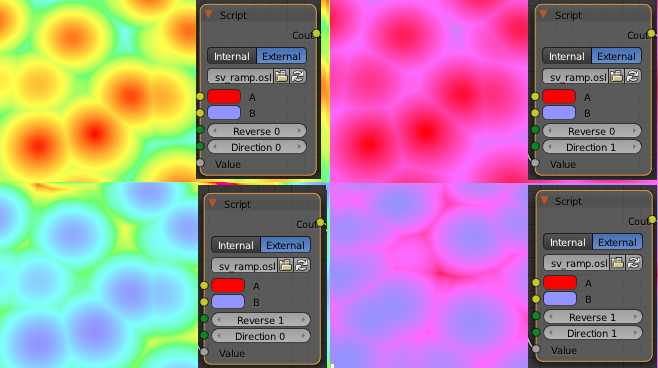# Hue Ramp OSL

Easily make an HSV ramp with two colours and option to reverse and/or change the direction.http://www.pasteall.org/38647/c

``````/* hue ramp by charlie */

color A = color(1,0,0),
color B = color(0,1,1),
int Reverse = 0,
int Direction = 0,
float Value = 1.0,
output color Cout = 0

)
{

string ColorSpace = "hsv";
color temp;
color Aspace = transformc("rgb",ColorSpace,A);
color Bspace = transformc("rgb",ColorSpace,B);

if (Direction) {
if(Aspace &lt; Bspace ) {
Aspace += 1.0;

}
}

if (Reverse) {
temp = mix(Bspace,Aspace,Value);
} else {
temp = mix(Aspace,Bspace,Value);
}

if (Direction) {
temp = mod(temp,1.0);
}

Cout = transformc(ColorSpace,"rgb",temp);

}
``````